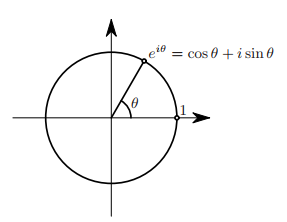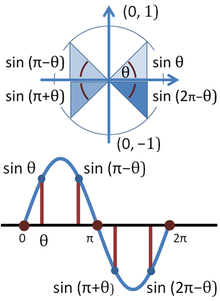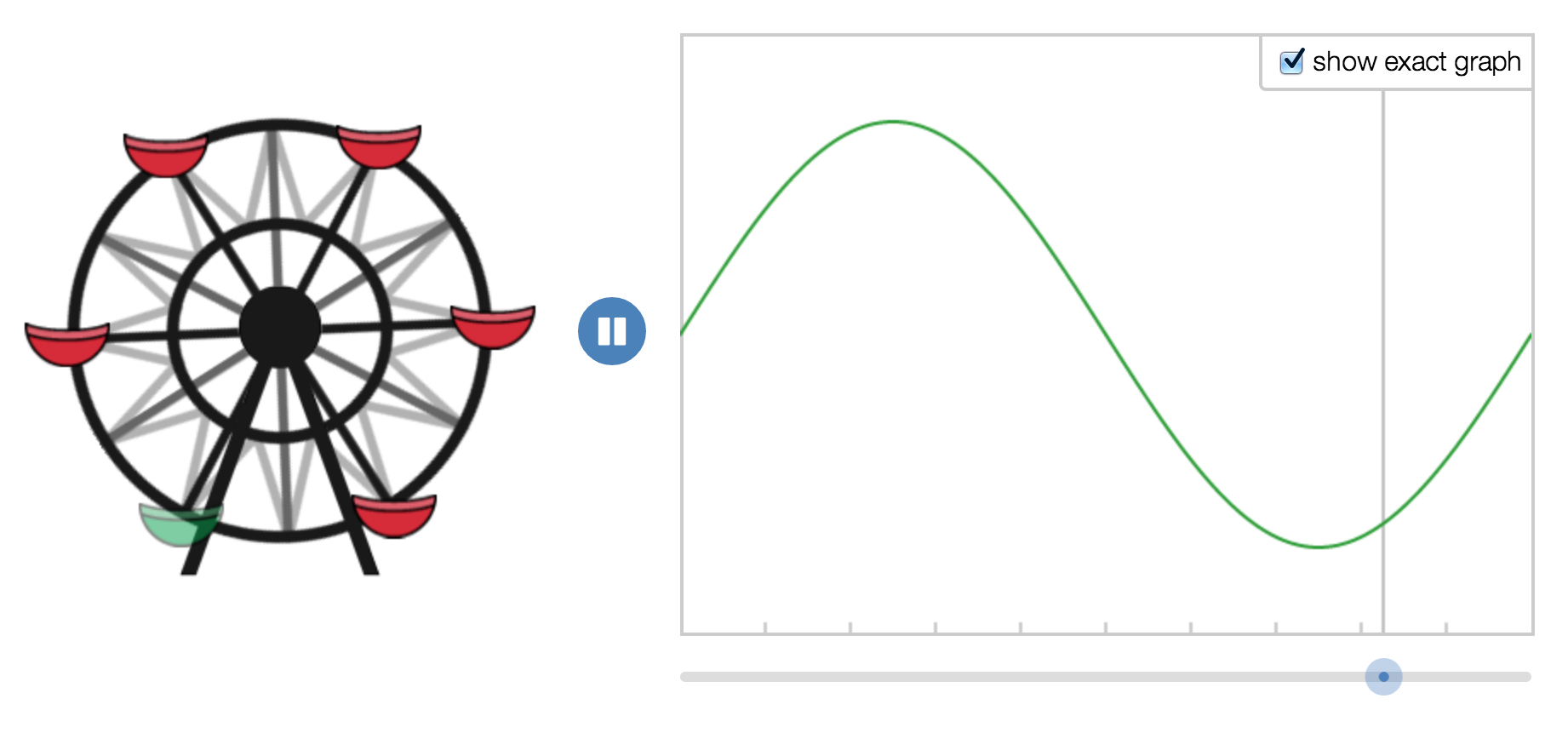# Trigonometry - (Cosine|Cosinus)

Cosine is the function of an angle (trigonometric function)

## Derivative

For the cosine function: $$f(x) = \cos x$$ The derivative is the sine: $$f'(x) = \sin x$$

## Animation

Recommended Pages
CSS - Function

A css function is a function that can be used in a CSS property value to calculate complex styling requirement. Css function are mostly used with CSS Variable. Below we define the space unit variable...Linear Algebra - (Dot|Scalar|Inner) Product of two vectors

A dot Product is the multiplication of two two equal-length sequences of numbers (usually coordinate vectors) that produce a scalar (single number) Dot-product is also known as: scalar product or...Mathematics - Complex Exponential (Euler's formula)

Euler's formula provides a powerful connection between analysis and trigonometry, and provides an interpretation of the sine and cosine functions (the sinusoidal functions) as weighted sums of the exponential...Statistics - (Normal|Gaussian) Distribution - Bell Curve

A normal distribution is one of underlying assumptions of a lot of statistical procedures. In nature, every outcome that depends on the sum of many independent events will approximate the Gaussian distribution...Trignometry - Identity function (sin2+cos2=1)

identify function in Trignometry where: Other illustration : The identity function is a derivative from the pythagorean...Trigonometry - (Sine|Sinus)

Sine is the function of an angle (trigonometric function). The word comes from the Latin sinus for gulf or bay, since, given a unit circle, it is the side of the triangle on which the angle opens. ...Trigonometry - Angle (or Arc) (Alpha - α)

(tri)angle Angle is counted in the Babylonian numeral system, base-60. (360 degrees in a circle) with the identity function - 360 degree degrees minutes seconds where:...Trigonometry - Function

Trigonometric functions are function of an angle. Trigonometric functions are important in the modeling of periodic phenomena. List: sine, cosine, tangent Trigonometric_functionsTrigonometry - Sinusoidal functions

A sinusoidal function is a time function and At the left, a cabin moving along a circle. At the right, the height of the cabin against time. ...